Properties of Metals, Stress-Strain & Elastic Constants - 1

# Properties of Metals, Stress-Strain & Elastic Constants - 1 - Civil Engineering SSC JE (Technical) - Civil Engineering (CE)

PROPERTIES OF METALS, STRESS - STRAIN AND ELASTIC CONSTANTS

PROPERTIES OF METALS:
1. Ductility:
-Ductility is the property by virtue of which material can be stretched to a reduced section under the action of tensile force. Large deformations are thus possible in ductile materials before the absolute failure or rupture takes place, some of the examples are mild steel, aluminium, copper, manganese, lead, nickel, brass, bronze, monel metal etc.

2. Brittleness:
-Brittleness is the lack of ductility i.e. material can not be stretched. In brittle materials, failure takes place with a relatively smaller deformation. This property is undesirable. For brittle materials fracture point & ultimate points are same, and after proportional limit very small strain is seen. Some of the examples are cast iron, concrete and glass.

-To distinguish between these two types of materials, materials with strain less than 5% at fracture point are regarded as brittle and those having strains greater than 5% at fracture point are called ductile. (This value for mild steel at fracture is about 25%).

3. Malleability:
-The property by which a material can be uniformly extended in all direction without rupture. A malleable material possess a high degree of plasticity. This property is of great use in operations like forging, hot rolling, drop (stamping) etc.

4. Toughness:
-The property which enables materials to absorb energy without fracture. This property is very desirable in case of cyclic loading or shock loading.

-The “Modulus of toughness” is measured as area under entire stress-strain curve and is the energy absorbed by material of the specimen per unit volume upto fracture stage.

Question for Properties of Metals, Stress-Strain & Elastic Constants - 1
Try yourself:Which property of a material allows it to be stretched to a reduced section under the action of tensile force?

Modulus of toughness: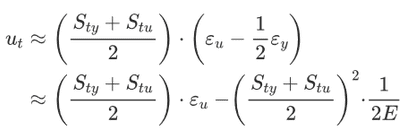Where,

Sty is the tensile yield strength,
Stu is the tensile ultimate strength,
εy is the strain at yield,
εu is the ultimate strain (total strain at failure), and
E is the elastic modulus.

• The modules of toughness will depend upon ultimate tensile strength and strain at failure (fracture strain). Hence the material which is very ductile, will exhibit a higher modulus of toughness as the case with mild steel.
• On the other hand the “Modulus of resilience” depends upon yield strength and hence a material with higher yield strength will have higher modulus of resilience.
• The ‘Modulus of resilience’ is the maximum elastic energy per unit volume that can be absorbed without attaining plastic stage.

Modulus of resilience:
μr=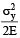Higher toughness a desirable property in materials used for gears, chains, crane hooks, freight car etc.
Higher resilience is desirable in springs.

5. Hardness:
-Hardness is defined as the resistance to indentation or scratching or surface abrasion.

Based upon this there are two methods of hardness measurement :
(i) Scratch hardness - Commonly measured by Mohr's test.
(ii) Indentation hardness (abrasion) measured by:

• Brinell hardness method
• Rockwell hardness
• Vickers hardness
• Knoop hardness
• It should be noted that ductile materials are tough and brittle materials are hard.

6. Fatigue:

The behaviour of material under variable loads (dynamic loads) is referred to as “fatigue”. In recent past several failures of structures have been noted due to fatigue.
Factors affecting fatigue are :
(iii) Corrosion
(iv) Temperature
(v) Stress concentration

7. Creep & Stress Relaxation:
-At any temperature, a material will progressively deform with the passage of time under constant loading, even if the stress is below yield point, this phenomenon is called creep. However such deformation is negligibly small at lower temperature.
-At higher temperature, due to greater mobility of atoms, most of the materials loose their strength and elastic constants also get reduced. Hence greater deformation at elevated temperature results even under constant loading. Therefore creep is more pronounced at higher temperature, hence it must be considered for design of engines & furnaces.
-The temperature at which the creep becomes very appreciable is half of the melting point temperature on absolute scale and is known as “Homologous temperature”.
-If a wire of metal is stretched between two immovable supports, so that it has an initial tension stress so. The stress in the wire gradually diminishes, eventually reaching a constant value. This process, which is a manifestation of creep, is called “Stress relaxation”.

Question for Properties of Metals, Stress-Strain & Elastic Constants - 1
Try yourself:Which property of a material is determined by the yield strength and represents the maximum elastic energy per unit volume that can be absorbed without attaining the plastic stage?

STRESS-STRAIN CURVE:
Simple tension test for mild steel :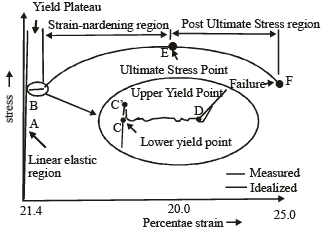• A is Limit of proportionality : Beyond A linear variation ceases.
• B is Elastic limit : The maximum stress up to which a specimen regains its original length on removal of applied load. For mild steel B is very near to A. for other materials B may be greater than A.
• C' is Upper yield point : The magnitude of the stress corresponding to C' depends on the cross-sectional area, shape of the specimen and the type of the equipment used to perform the test. It has no practical significance. 
• C is lower yield point : The stress at C is the yield stress (σy) with a typical value of σy = 250 N/mm2 for mild steel. The yielding begins at this stress.
• CD represents perfectly plastic region : It is the strain which occurs after the yield point C, without any increase in stress. The strain corresponding to point D is about 1.4% and corresponding to C is about 0.12% for mild steel. Hence plastic strain is 10 to 15 times of elastic strain.
• DE represents strain hardening : In this range further addition of stress gives additional strain, however strain increases with faster rate in this region. The material in this range undergoes change in its atomic and crystalline structure, resulting in increased resistance to further deformation. This portion is not used for structural design.
• E is Ultimate point : The stress corresponding to this point is ultimate stress (σy) and the corresponding strain is about 20% for mild steel.
• F is fracture point : Stress corresponding to this is called breaking stress & strain is called fracture strain.
• It is about 25% for mild steel.
• Region between E & F is the necking region, in which area of cross-section is drastically decreased.

Important Points to be noted :

1. Strain that occurs before the yield point is called elastic strain and that which occurs after yield point with no increase in stress is called plastic strain. For mild steel, Plastic Strain = 10 to 15 times of elastic strain

2. Ideal curve for tension is shown, however actual behaviour is different and indicates apparently reduced yield stress in compression for mild steel. The divergence between tension and compression results it is explained by Bauchinger and is called “Bauchinger effect”.

3. The stress is defined as the ratio of load to original area (Ao) It is also known as ‘engineering stress’ or ‘stress’ or ‘nominal stress’.
Engg. stress ‘or’ Nominal stress =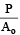On the contrary when changing area is taken into account, the ratio of load to actual area (A) is called ‘true stress’.
True stress = P/A

SIMPLE STRESS AND STRAIN:
-It is the internal resistance offered by the body against external loading or deformation. Stresses may be of following type :
(i) Direct stresses or normal stresses which may be tensile or compressive.
(ii) Shear or Tangential stresses.
(iii) Transverse or Bending stresses.
(iv) Torsional or Twisting stresses.
-For direct stresses, if area under consideration is original area, then it is known as engineering stress or nominal stress or simply stress. But, if the area taken is actual area, then stress is known as true stress.

Nominal Stress =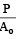here, Ao = Original area of specimen
A = Actual area of specimen
-When a prismatic bar is subjected to axial load, it undergoes a change in length. This change is length is usually called deformation. The deformation per unit length of the bar is termed as strain. Since strain is deformation per unit length, it is a dimensionless quantity. But sometimes in practice, strain is recorded in forms such as mm/m or µm/m etc.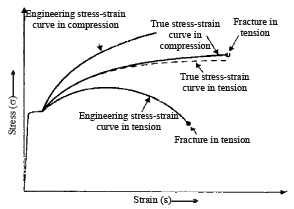Tension and Compression Monotonic Stress-strain Diagrams: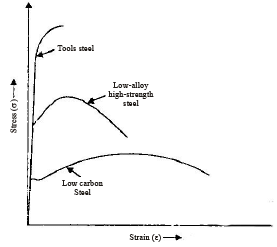Tensile stress-strain diagram for different steels: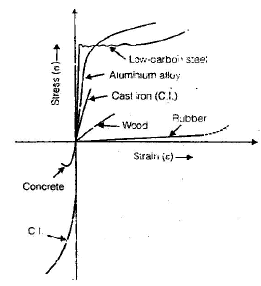Stress-strain diagrams for different materials:

1. Hooke’s Law
-It states that within elastic limit stress for most of the metals is proportional to strain.

Stress ∝ Strain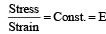Where, E = Young’s modulus of elasticity.
-Hooke’s law is valid up to limit of proportionality. However for mild steel proportional limit and Elastic limit are almost equal, but for other metals & materials elastic limit may be higher than proportional limit, for example-rubber.
-The slope of stress-strain curve is called modulus of elasticity (E). The modulus of elasticity (E) is the constant of proportionality which is defined as the intensity of stress that causes unit strain. Thus, modulus of elasticity (E) has the units same as units of stress.

2. Proof Stress:
-When a material such as aluminium does not have an obvious yield point and yet undergoes large strains after the proportional limit is exceeded, an arbitrary yield stress may be determined by the offset method.
-A line parallel to initial linear part is drawn, which is offset by some standard amount of strain such as 0.2%. The intersection of the offset point (A) defines the yield stress of offset yield stress, which is slightly above the proportional limit and is called proof stress.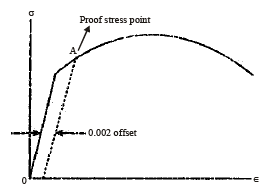Stress-strain curve for ‘aluminium’

The document Properties of Metals, Stress-Strain & Elastic Constants - 1 | Civil Engineering SSC JE (Technical) - Civil Engineering (CE) is a part of the Civil Engineering (CE) Course Civil Engineering SSC JE (Technical).
All you need of Civil Engineering (CE) at this link: Civil Engineering (CE)

## FAQs on Properties of Metals, Stress-Strain & Elastic Constants - 1 - Civil Engineering SSC JE (Technical) - Civil Engineering (CE)

 1. What are the properties of metals?Ans. Metals have several distinct properties that set them apart from other materials. Some common properties of metals include high electrical and thermal conductivity, malleability, ductility, and opacity. Metals are also typically shiny in appearance and have a high melting and boiling point.
 2. What is stress-strain relationship in metals?Ans. Stress-strain relationship in metals refers to the correlation between the applied stress (force per unit area) and the resulting strain (deformation) in a metal material. When a metal is subjected to stress, it undergoes deformation, and the extent of this deformation is measured by strain. The stress-strain relationship helps determine the mechanical properties of a metal, such as its elastic modulus, yield strength, and ultimate tensile strength.
 3. What are elastic constants in metals?Ans. Elastic constants in metals are numerical values that represent the material's response to external forces or stresses. The three main elastic constants used to describe the behavior of metals are Young's modulus (E), shear modulus (G), and bulk modulus (K). Young's modulus measures the material's stiffness, shear modulus quantifies its resistance to shearing forces, and bulk modulus represents its resistance to volume change under pressure.
 4. How are stress and strain related in metals?Ans. Stress and strain are related in metals through Hooke's Law, which states that stress is directly proportional to strain within the elastic limit of the material. Mathematically, this relationship can be expressed as stress = elastic modulus × strain. The elastic modulus, such as Young's modulus, determines how much stress a material will experience for a given amount of strain.
 5. What is the significance of stress-strain curves in studying metals?Ans. Stress-strain curves provide valuable information about the mechanical behavior of metals. These curves depict the relationship between stress and strain during the deformation of a material. By analyzing the shape of the curve, important properties of metals can be determined, including their elastic limit, yield strength, ultimate tensile strength, and ductility. The stress-strain curve also helps identify the material's ability to withstand external forces before failure occurs, making it a crucial tool in material testing and design.

## Civil Engineering SSC JE (Technical)

2 videos|122 docs|55 tests

## Civil Engineering SSC JE (Technical)

2 videos|122 docs|55 tests
Signup to see your scores go up within 7 days! Learn & Practice with 1000+ FREE Notes, Videos & Tests.
10M+ students study on EduRev
Track your progress, build streaks, highlight & save important lessons and more!(Scan QR code)
Related Searches

,

,

,

,

,

,

,

,

,

,

,

,

,

,

,

,

,

,

,

,

,

,

,

,

;